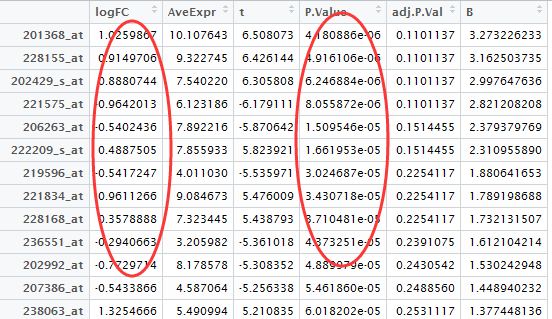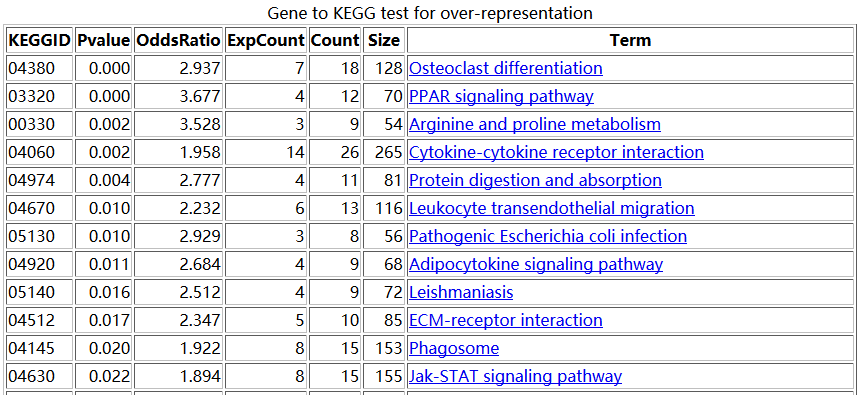# 用超几何分布检验做富集分析

setwd("D:\\my_tutorial\\补\\用limma包对芯片数据做差异分析")

View(DEG)probeset=rownames(DEG[abs(DEG[,1])>0.5 & DEG[,4]<0.05,])

library(hgu133plus2.db)

library(annotate)

platformDB="hgu133plus2.db";

EGID <- as.numeric(lookUp(probeset, platformDB, "ENTREZID"))

length(unique(EGID))

# 775

diff_gene_list <- unique(EGID)

library(GOstats)

library(org.Hs.eg.db)

#then do kegg pathway enrichment !

hyperG.params = new("KEGGHyperGParams", geneIds=diff_gene_list, universeGeneIds=NULL, annotation="org.Hs.eg.db",

categoryName="KEGG", pvalueCutoff=1, testDirection = "over")

KEGG.hyperG.results = hyperGTest(hyperG.params);tmp=toTable(org.Hs.egPATH)
GeneID2Path=tapply(tmp[,2],as.factor(tmp[,1]),function(x) x)
Path2GeneID=tapply(tmp[,1],as.factor(tmp[,2]),function(x) x)
#phyper(k-1,M, N-M, n, lower.tail=F)
#n*M/N
diff_gene_has_path=intersect(diff_gene_list,names(GeneID2Path))
n=length(diff_gene_has_path) #321 # 这里算出你总共抽取了多少个球
N=length(GeneID2Path) #5870  ##这里算出你总共有多少个球（这里是错的，有多少个球取决于背景基因！一般是两万个）
options(digits = 4)
for (i in names(Path2GeneID)){
M=length(Path2GeneID[[i]])  ##这个算出你的所有的球里面，白球有多少个
exp_count=n*M/N  ###这里算出你抽取的球里面应该多多少个是白色
k=0         ##这个k是你实际上抽取了多少个白球
for (j in diff_gene_has_path){
if (i %in% GeneID2Path[[j]]) k=k+1
}
OddsRatio=k/exp_count
p=phyper(k-1,M, N-M, n, lower.tail=F)  ##根据你实际上抽取的白球个数，就能算出富集概率啦！
print(paste(i,p,OddsRatio,exp_count,k,M,sep="    "))
}